SPSS TUTORIALS BASICS ANOVA REGRESSION FACTOR CORRELATION

# Sampling in SPSS – Quick Tutorial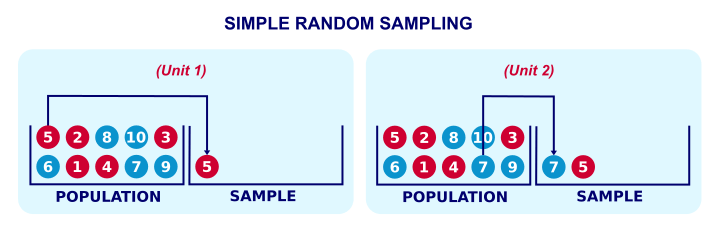How to draw one or many samples from your data in SPSS? This tutorial demonstrates some simple ways for doing so. We'll point out some tips, tricks and pitfalls along the way.
Let's get started and create some test data by running the syntax below.

## SPSS Syntax for Creating Test Data

*Create test data with 100 cases.

data list free/id.
begin data
0 0 0 0 0     0 0 0 0 0         0 0 0 0 0     0 0 0 0 0         0 0 0 0 0     0 0 0 0 0         0 0 0 0 0     0 0 0 0 0         0 0 0 0 0     0 0 0 0 0
0 0 0 0 0     0 0 0 0 0         0 0 0 0 0     0 0 0 0 0         0 0 0 0 0     0 0 0 0 0         0 0 0 0 0     0 0 0 0 0         0 0 0 0 0     0 0 0 0 0
end data.

compute id = \$casenum.
execute.

## Result

We now have 1 variable and 100 cases. For each case, our variable contains \$casenum. The screenshot below shows the last handful of cases in our data.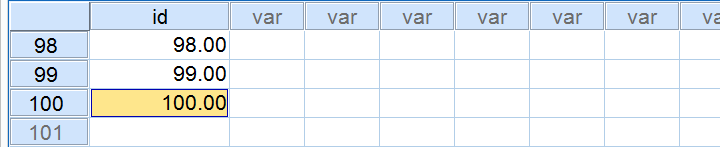## Simple Sampling Without Replacement

Simple random sampling means that each unit in our population has the same probability of being sampled. “Without replacement” means that a sampled unit is not replaced into the population and thus can be sampled only once. The figure below illustrates the process.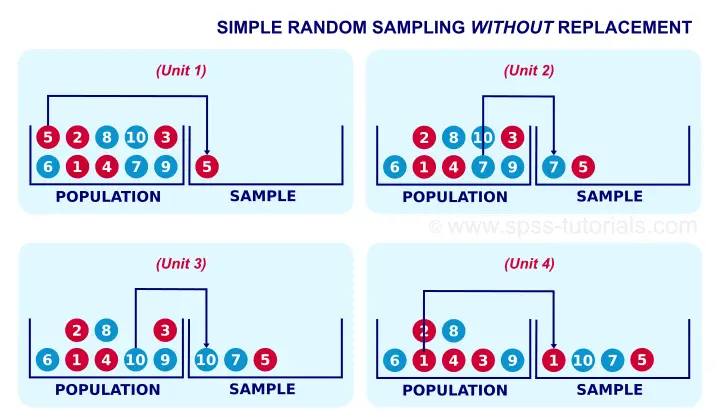Simple random sampling without replacement is the easiest option for sampling in SPSS. The syntax below shows the first option for doing so.

## Simple Random Sampling without Replacement - Example I

*1. Render sampling process replicable.

set rng mc seed 1.

*2. Draw sample.

sample 20 from 100.
execute.

## Result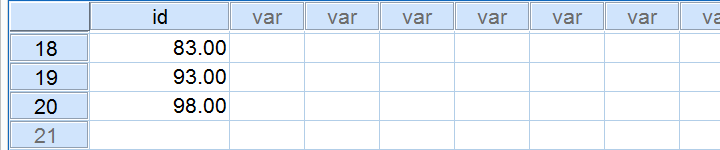## Notes

This first example is the easiest way to draw just one sample when we know the number of cases in our data (100 in our example). Note that we have 20 cases left after running it.
If we rerun our sampling syntax, we usually want the exact same random sample to come up. One way for ensuring this is running SET RNG MC SEED 1. just prior to sampling.

## Simple Random Sampling without Replacement - Example II

Let's first rerun our test data syntax. Next, the syntax below shows a second option for sampling without replacement.

*1. Compute random numbers between 0 and 1.

compute s1 = rv.uniform(0,1).

*2. Rank random numbers.

rank s1.

*3. Select 20 cases with lowest random numbers.

select if (rs1 <= 20).
execute.

## Notes

As we'll see later on, this second example is a first step towards repeated sampling and stratified random sampling. On top, it doesn't require knowing how many cases we have in our data.

## Simple Random Sampling without Replacement - Example III

Again, we'll rerun our test data syntax, followed by the syntax below.

*1. Compute random numbers between 0 and 1.

set seed 1.

compute s1 = rv.uniform(0,1).

*2. Rank random numbers.

rank s1.

*3. Recode rank variable into filter variable.

recode rs1 (lo thru 20 = 1)(else = 0).

*4. Switch filter on.

filter by rs1.

*5. Inspect output.

descriptives id.

## Result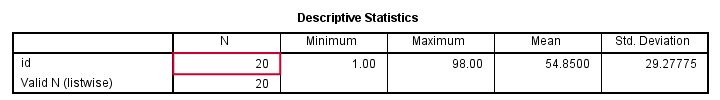## Notes

This third examples uses FILTER instead of deleting unsampled cases with SELECT IF. This leaves all our cases -including a variable that indicates our sample- nicely intact in our data. As shown below, the strikethrough in data view as well as the status bar tell us that a filter is actually in effect.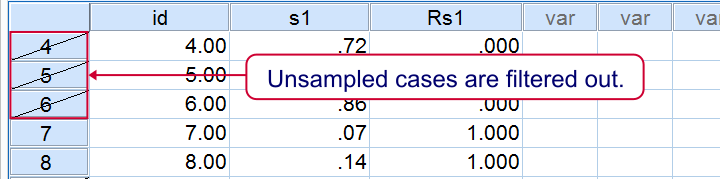## Repetitive Sampling in SPSS

Repeated random sampling is the basis for most simulation studies. We presented such simulations for explaining the basic idea behind ANOVA and the chi-square test.
Simulation studies usually require looping over SPSS procedures, which are basically commands that inspect all cases in our dataset. The right way for doing so is with Python as shown in the syntax below. Running it requires the SPSS Python Essentials to be properly installed.

## SPSS Repeated Sampling with Python Syntax

*Requires SPSS Python Essentials: draw 10 samples of 20 cases and compute descriptives on each.

begin program.
import spss
for sample in range(10):
spss.Submit('''
temporary.
sample 20 from 100.
descriptives id.
''')
end program.

## Notes

We use TEMPORARY here for drawing our repeated samples. Note that we're basically simulating a sampling distribution over mean scores here. These mean scores (over 20 cases each) will be roughly normally distributed due to the central limit theorem.

## SPSS Repeated Sampling Example 2

The syntax below uses a different approach for repeated sampling that'll be the basis for simple random sampling with replacement later on. All sample variables will be left in our data -a feature we may or may not like.

## SPSS Repeated Sampling with Python Syntax

*1. Create 10 variables with random numbers.

do repeat #s = s1 to s10.
compute #s = rv.uniform(0,1).
end repeat.

*2. Rank previous variables.

rank s1 to s10.

*3. Convert rank variables into filter variables.

recode rs1 to rs10 (lo thru 20 = 1)(else = 0).

*4. Run filtered descriptives.

begin program.
import spss
for sample in range(1,11):
spss.Submit('''
filter by rs%d.
descriptives id.
'''%sample)
end program.

*5. Switch off filter.

filter off.

## Simple Sampling With Replacement

Strictly, most inferential statistics quietly assume that our data are obtained by simple random sampling with replacement. A textbook example is drawing a marble from a vase, writing down its color and putting it back into the vase before sampling a second (third...) marble. Like so, each marble may be sampled several times. The figure below illustrates the idea.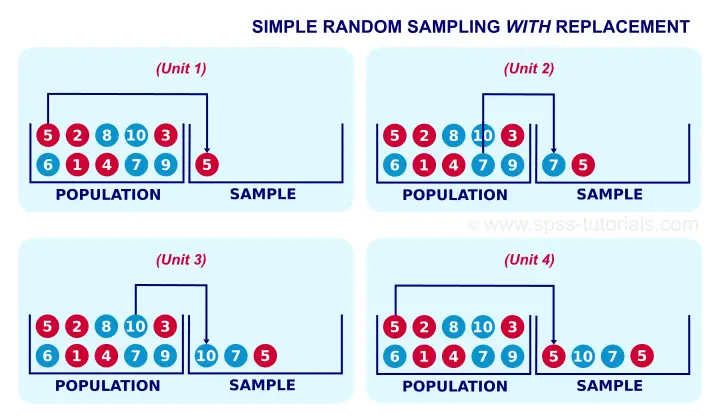In real-world research, simple sampling with replacement is not common. This is because respondents who are “sampled” a second or third time
will probably just tell the statistician to “f@#k off”.
Anyway. The syntax below demonstrates simple random sampling with replacement in SPSS. It uses both WEIGHT and FILTER in order for the sample to take effect.

## Simple Random Sampling With Replacement Syntax

*1. Sampling 50 out of 100 cases with replacement.

set seed 1.

do repeat #s = s1 to s50.
compute #s = rv.uni(0,1).
end repeat.

*2. Rank random numbers.

rank s1 to s50.

*3. Each rank variable represents a single draw with replacement.

recode rs1 to rs50 (2 thru hi = 0).

*4. The sum of our 50 draws is our sample variable.

compute sample = sum(rs1 to rs50).

*5. Inspect sample variable in data view.

sort cases by sample (d).

*6. Sample variable should sum to n = 50 cases.

descriptives sample/statistics min max mean sum.

*7. Filter not necessary but circumvents warning when WEIGHT is used.

filter by sample.

*8. Use sample as weight variable.

weight by sample.

*9. Descriptives on 50 cases.

descriptives id.

## Result in Data View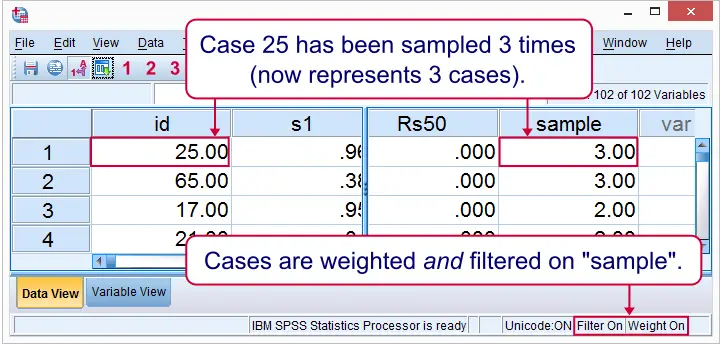Right. So those are some basics on sampling in SPSS. I hope you found them helpful.

In any case, thanks for reading and keep up the good work!

# Tell us what you think!

*Required field. Your comment will show up after approval from a moderator.

# THIS TUTORIAL HAS 7 COMMENTS:

•### By Ruben Geert van den Berg on June 30th, 2020

Hi Justin!

I recently developed a non Python solution for this that may work (much) better:

-create x random variables for x samples
-RANK these x random variables
-for each random variable, copy your outcome variable if (RANK <= N) where N is the desired sample size -if (RANK > N), make sure the copy is \$SYSMIS
-now a single DESCRIPTIVES table summarizes the results

Alternatively, you can create results with AGGREGATE as a dataset. If you FLIP. this dataset, the statistics thus created become cases rather than variables. You can no even run a histogram over them.

See if you can make this work too. I didn't find it too hard but it was nice and fast because it doesn't require a lot of data passes.

If you really can't make it work, I can see if I can find my syntax and perhaps send it by email, ok?

Kind regards!

Ruben
SPSS tutorials

•### By Justin on June 30th, 2020

Hi Ruben!

Many thanks for your help here. I gave both of these a try, but wasn't able to get either working from the descriptions alone. If you could send the syntax for these steps I'm confident I could get it working.

Thanks kindly for your help and the excellent site!

Justin